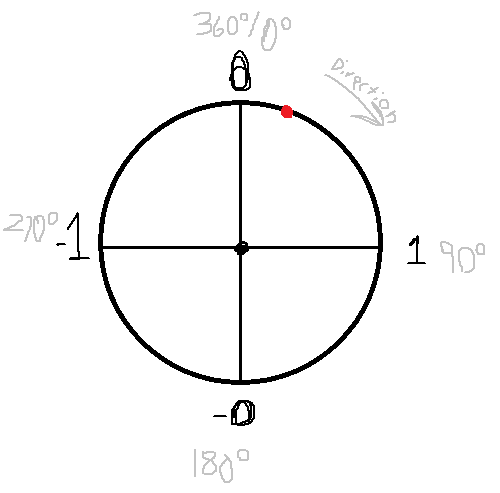# Can anyon explain to me how this script works?

I was trying to figure out how to make a part circle another part, and I eventually found a script online that allows you to do this. I’m not sure how it works though, and I would be happy if anyone could explain to me how this script allows parts to circle around other parts in a perfect circle.

``````local MainPart = script.Parent
local Part = workspace.Part
local theta = 0
local radius = (Part.Position - MainPart.Position).Magnitude

Part.Position = Vector3.new(MainPart.Position.X, Part.Position.Y, MainPart.Position.Z + radius);
while wait() do
theta +=0.1;
end
``````
2 Likes

The important bit is `math.sin` and `math.cos`. These are trigonometry functions that allow you to create a unit circle. (unit in mathematics means it has a distance/magnitude of 1)

`math.cos(angle)` gives you the x position of a point on a circle at `angle`.
`math.sin(angle)` gives you the y position. In the code `sin` and `cos` are switched around, that only means that the angle is going to be 90 degrees rotated.

Since this creates a unit circle (magnitude of 1), we need to multiply the resulting x and y by the radius of our circle. This gives us the true coordinates of a point on the circle at an angle and radius. Then you can add an X and Y position to change the location of the circle.

3 Likes

So, what you see here is apart of trigonometry. I personally don’t know it full well to use it with a lot of my code, but your script simplifies the uses of such phenomenon.

I’ll try my best to give a brief definition of the trigonometry. So, from how I could understand it, it deals with the uses of the quotient of two sides of a right triangle. And dividing the different sides in different ways can create different results, which those division formulas have names.

And `math.sin` is a part of those formulas, named Sine, which uses the formula `Opposite / Hypotenus`. This function/formula is how you’re able to get the perfect angle of a circle without having to do any more complicated calculations. The theta is there as the degrees that the sin needs to convert into radians. If you don’t know what radians are, I suggest you search them up to understand them better… But the basic idealogy is that they turn degrees into a unit (it’ll go to 1).

So, since the theta started at 0 (well, 0.1 since you’ve added it first), the math.sin would return 0.1. But when it goes from 90 and continues, it’ll then start decreasing to 0 again. I would have a trouble time explaining why it does that, so here’s an image to demonstrate.This is a unit circle. So, basically, 90 degrees is a whole one in the unit circle; the quarter of a circle. Meaning, everytime you increase the number (`theta` in this case), you’re going around that unit circle… eventually making it back to the 0. This, right here, is why you’re able to make a perfect circle.

And the radius is there if you want a desired radius. So, yeah, I hope this helps!

I’ll link to some more useful resources if this failed to help you. Again, I suck at explaining some things and I know there’s other people out there that definitely is better at explaining this so. Here’s some links:

1 Like

This sounds a lot more readable and understandable compared to what I said ealier, really nice job.

1 Like

Do you know why whenever I move the Main Parts position manually causes the part rotating to rotate further away from the Main Part?

You mean while it’s running? I don’t. If you move them further away from each other before the script starts though, then it will change the radius because the radius is calculated by the distance between them when the script first starts.